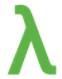Net-informations.com

# What is lambda in Python?

Lambda, the 11th letter of the Greek alphabet , is the symbol for wavelength . Lambda comes from the Lambda Calculus and refers to anonymous functions in programming.In Python, Lambda is an expression . Lambda's body is a single expression, not a block of statements. Because it is limited to an expression, a lambda is less general than a def you can only squeeze so much logic into a lambda body without using statements such as if. This is not exactly the same as lambda in functional programming languages, but it is a very powerful concept that's well integrated into Python and is often used in conjunction with typical functional concepts like map() , filter() and reduce() . Moreover, Lambda can be used wherever function objects are required.

The general syntax of a lambda function is quite simple:

The argument list consists of a comma separated list of arguments and the expression is an arithmetic expression using these arguments.

Here's an example. You can build a function in the normal way, using def, like this:

Using lambda:

#### example

Lambda allows you to write quick throw away functions without naming them. It also provides a nice way to write closures also.

#### example

Find the sum of two numbers using lambda

#### output

Lambda in Conditional expressions:

result = lambda x: "Bigger than 100" if x > 100 else "Smaller than 100"

print(result(99))

### Calculating the sum of the numbers from 1 to 1000 using lambda

#### output

net-informations.com (C) 2022    Founded by raps mk